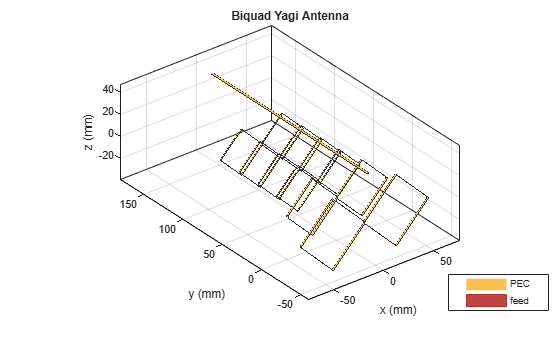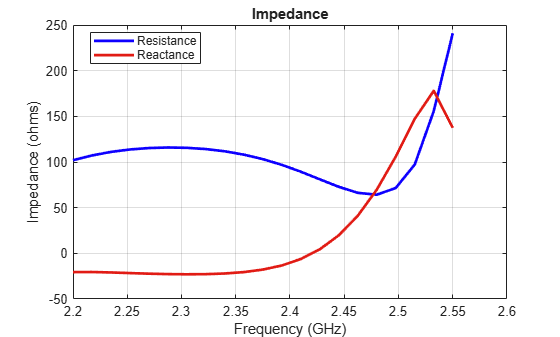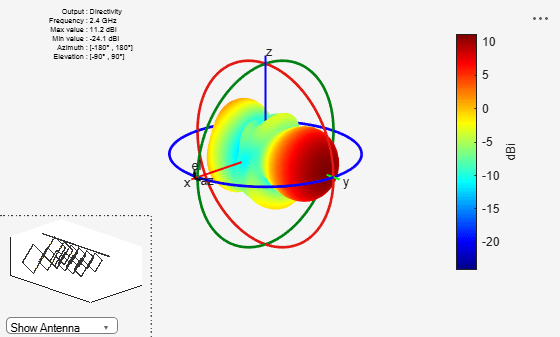Main Content

# Analysis of biquad Yagi for WIFI applications

This example shows how to analyze the performance of a customized Yagi-Uda antenna. Biquad Yagi antenna is popularly used in WIFI applications.

### Define parameters

Design the biquad yagi antenna to operate at 2.4GHz. Use dimensions of 30mm element for the first parasitic element followed by 31mm, 32mm, 33mm, then 34mm driven element and a 36mm reflector at the rear. Define the design parameters of the antenna as provided.

```ref = biquad('Tilt',90,'ArmLength',36e-3); % Reflector exct = biquad('Tilt',90,'ArmLength',34e-3); % Driven element direct1 = biquad('Tilt',90,'ArmLength',33e-3); % Director1 direct2 = biquad('Tilt',90,'ArmLength',32e-3); % Director2 direct3 = biquad('Tilt',90,'ArmLength',31e-3); % Director3 direct4 = biquad('Tilt',90,'ArmLength',30e-3); % Director4 ```

### Create a biquad yagi antenna

Space the parasitic elements 17mm from driven element and the reflector 19mm from the driven element. You can increase and decrease the length of the Boom and, you can move the Boom by changing the BoomOffset property. Create a quadCustom antenna using the parameters defined.

```ant = quadCustom('Exciter',exct,'Director',{direct1,direct2,direct3,direct4},... 'DirectorSpacing',17e-3,'Reflector',{ref},'ReflectorSpacing',19e-3,... 'BoomOffset',[0 0.03 0.030],'BoomLength',0.09); figure; ant.Tilt = 180; ant.TiltAxis = [0 1 1]; show(ant); % view(-13,17); title('biquad yagi Antenna'); ```### Calculate the antenna impedance

Calculate the antenna impedance over the frequency range of 2.3GHz to 2.6GHz. From the figure, observe the antenna resonates around 2.4GHz.

```figure; impedance(ant, linspace(2.2e9, 2.75e9, 31)); ```### Plot Reflection Coefficient

Plot the reflection coefficient for this antenna over the band and a reference impedance of 50 ohms.

```figure; s = sparameters(ant, linspace(2.2e9, 2.75e9, 31)); rfplot(s); ```### Calculate and plot pattern

Plot the radiation pattern for this antenna at the frequency of best match in the band.

```figure; pattern(ant,2.45e9); ```### Current distribution

```figure; current(ant, 2.45e9,'scale','log10'); ```## Support

#### Hybrid Beamforming for Massive MIMO Phased Array Systems

Download the white paper